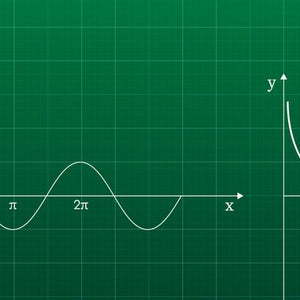# 91028 Relationships between Tables, Equations and Graphs

• \$14.95

### What you'll learn

This course covers the things you'll need to know and be able to do for achievement standard 91028.

In it you'll find answers to questions like:

• How do we solve linear equations and inequations, and simultaneous equations with two unknowns?
• How do we relate rate of change to the gradient of a graph?
• How do we solve quadratic equations?
• How do we solve simple exponential equations?
• How can we find optimal solutions, using numerical approaches?
• How can we relate graphs, tables and equations to simple exponential relationships found in number and spatial patterns?

### How you'll learn

People learn in different ways. This resource contains a range of images, videos and interactive activities to help you learn. You will also find activities designed specifically for you. These activities use an artificial intelligence (AI) tutor called Amy.

Self-paced learning – There are ‘suggested’ times for each page - these are just a guideline. You might find that you are able to move quickly through some activities, and others take longer. Plan your learning to suit you.

Amy activities - Throughout this course there are activities provided by Amy.  Amy's activities are designed to take you step-by-step through each question so that you can show you understand the steps in each process.  Each Amy activity box will give you five sets of questions.  When you've mastered the concept, you'll receive a message that says: "Well done.  You have finished this assignment."  Amy knows what you've done before so she'll learn what questions you need.